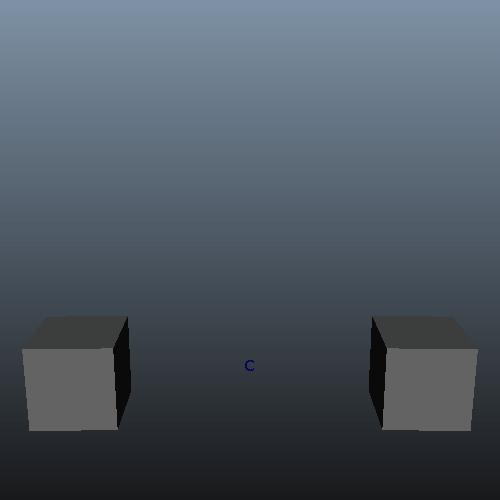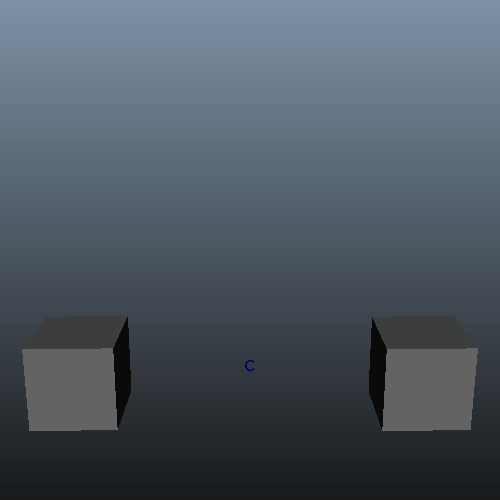TLDR:
Exporting deformer weights using `cmds.deformerWeights` only works for deformers influencing one object.
Except with a regular expression in the skip argument to skip anything except the imported deformer name.

Set up the example scene: Two poly cubes being 100% influenced by one cluster. The cluster moves up and down to visualize the influence.

```cmds.file(f=True, new=True)
# create 2 cubes
cube1 = cmds.polyCube(ch=False)
cmds.move(-2, 0, 0)
cmds.rotate(0, -90, 0)
cube2 = cmds.polyCube(ch=False)
cmds.move(2, 0, 0)
cmds.rotate(0, -90, 0)
# create a cluster that affects both cubes
cmds.select(cube1, cube2)
clu, cluHandle = cmds.cluster()
cmds.setKeyframe(cluHandle, at='ty', time=0, value=0)
cmds.setKeyframe(cluHandle, at='ty', time=24, value=0.5)
cmds.setKeyframe(cluHandle, at='ty', time=48, value=0)
```Let’s set some weights we can export.

```# weights the verts 0-3 on cube1 and 4-7 on cube2 to 0
for vertexNo in range(4):
cmds.percent(clu, '%s.vtx[%s]' % (cube1, vertexNo), value=0)
cmds.percent(clu, '%s.vtx[%s]' % (cube2, 7-vertexNo), value=0)

```

Now the inner parts of cube1 and parts of cube2 move with the cluster.If we export these weights the regular way we only get the weights for cube1

```cmds.deformerWeights('weightsRegular_%s.xml' % clu, path='/tmp', deformer=clu, ex=True)

# Let's test the export:
with open('/tmp/weightsRegular_%s.xml' % clu, 'r') as fh:
for line in fh:
print line,
```

Only the weights for cube1, sadly

```

```

What happens if we import this?

```# set all weights to 0 before importing
for vertexNo in range(7):
cmds.percent(clu, '%s.vtx[%s]' % (cube1, vertexNo), value=0)
cmds.percent(clu, '%s.vtx[%s]' % (cube2, vertexNo), value=0)

cmds.deformerWeights('weightsRegular_%s.xml' %clu, path='/tmp', deformer=clu, im=True)
```

The same weights were imported onto cube1 and cube2 because only cube1 was exportedHow do we fix this? We use a regular expression with the skip argument to skip anything except the exported deformer name:

```# restore all weights to before exporting again
for vertexNo in range(7):
cmds.percent(clu, '%s.vtx[%s]' % (cube1, vertexNo), value=1)
cmds.percent(clu, '%s.vtx[%s]' % (cube2, vertexNo), value=1)
for vertexNo in range(4):
cmds.percent(clu, '%s.vtx[%s]' % (cube1, vertexNo), value=0)
cmds.percent(clu, '%s.vtx[%s]' % (cube2, 7-vertexNo), value=0)

# export weights for clu on cube1 AND cube2
# we use a regular expression with the skip argument to skip anything except the exported deformer name
cmds.deformerWeights('weightsSkip_%s.xml' %clu, path='/tmp', deformer=clu, ex=True, skip=('?!%s' % clu))
```

Let’s test the export:

```with open('/tmp/weightsSkip_%s.xml' % clu, 'r') as fh:
for line in fh:
print line,
```

Now we’ve got the weights for cube1 AND cube2

```

```

Let’s test by importing the weights. Again we use a regular expression with the skip argument to skip anything except the imported deformer name.

```# set all weights to 0 before importing
for vertexNo in range(7):
cmds.percent(clu, '%s.vtx[%s]' % (cube1, vertexNo), value=0)
cmds.percent(clu, '%s.vtx[%s]' % (cube2, vertexNo), value=0)

# import weights for clu back on cube1 AND cube2
# again we use a regular expression with the skip argument to skip anything except the imported deformer name
cmds.deformerWeights('weightsSkip_%s.xml' % clu, path='/tmp', deformer=clu, im=True, skip=('?!%s' % clu))
```

And that’s how it’s supposed to look. Exactly like what we exported.You might be interested in